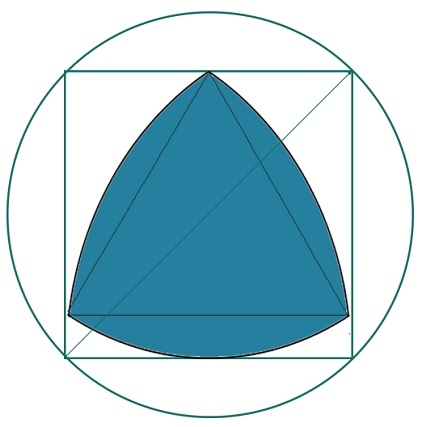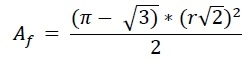# Biggest Reuleaux Triangle within a Square which is inscribed within a Circle?

Here we will see the area of biggest Reuleaux triangle inscribed within a square, That square is inscribed inside one circle. The side of the square is ‘a’. The radius of the circle is ‘r’. As we know that the diagonal of the square is the diameter of the circle. So −

2𝑟 = 𝑎√2
𝑎 = 𝑟√2

And the height of the Reuleaux triangle is h.The height of the Reuleaux triangle is same as a. So a = h. So the area of Reuleaux triangle is −## Example

#include <iostream>
#include <cmath>
using namespace std;
float areaReuleaux(float r) { //radius of ciecle is r
if (r < 0) //if a is negative it is invalid
return -1;
float area = ((3.1415 - sqrt(3)) * (r * sqrt(2)) * (r * sqrt(2)))/2;
return area;
}
int main() {
}
Area of Reuleaux Triangle: 50.7402